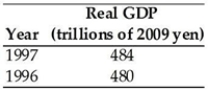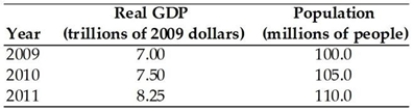# Macroeconomics Australia Study Set 1

## Quiz 8 : Economic GrowthStudy FlashcardsLooking for Economics Homework Help?

## Quiz 8 :Economic Growth

Question TypeReal GDP per person averaged $150 a year (in 2009 dollars) from 1,000,000 BC until 1620. Then in ________ real GDP began to increase without limit and by 1850 had risen to twice its 1650 level because ________. Free Multiple Choice Answer: Answer: E Tags Choose question tagFor the world, what period of time experienced the fastest growth rate of real GDP per person? Free Multiple Choice Answer: Answer: C Tags Choose question tagIf a country experiences a real GDP growth rate of 1 per cent and population growth of 2 per cent, then the growth rate of real GDP per person is Free Multiple Choice Answer: Answer: E Tags Choose question tagWhich of the following variables is used to determine a country's economic growth? i. Real GDP ii. Wages iii. Inflation Multiple Choice Answer: Tags Choose question tag-The table above gives information about the economy of Japan. The economic growth rate in 1997 was ________ per cent. Multiple Choice Answer: Tags Choose question tag-Using the data in the table above, real GDP per person in 2009 is Multiple Choice Answer: Tags Choose question tagEconomic growth is defined as Multiple Choice Answer: Tags Choose question tagIf real GDP in year 1 is$72 million and real GDP in year 2 is $87 million, then the growth rate of real GDP is Multiple Choice Answer: Tags Choose question tagAccording to the Rule of 70, if a country grows at 2.0 per cent per year instead of 1.5 per cent per year, how years less will it take to double its level of real GDP? Multiple Choice Answer: Tags Choose question tagIf it took 20 years for real GDP to double, what was the growth rate of real GDP? Multiple Choice Answer: Tags Choose question tagThe population in the current year is 31.5 million and the real GDP is$814 million. The previous year's statistics were a population of 31 million and a real GDP of $800 million. The change in the standard of living, measured by growth in real GDP per person, is Multiple Choice Answer: Tags Choose question tagIf real GDP grows at a faster rate than population, then the standard of living, as measured by real GDP per person, Multiple Choice Answer: Tags Choose question tag-Using the data in the table above, the growth rate of real GDP has Multiple Choice Answer: Tags Choose question tagApproximately how long will it take Ethiopia to double its real GDP per person of$100 if its growth rate of real GDP per person is 0.9 per cent?
Multiple Choice
Tags
Choose question tag-Using the data in the table above, the growth rate of real GDP for 2010 is equal to
Multiple Choice
Tags
Choose question tagEconomic growth is a sustained expansion of production possibilities measured as the increase in ________ over a given period.
Multiple Choice
Tags
Choose question tagThe Rule of 70 states that the level of a variable will double in
Multiple Choice
Tags
Choose question tagA nation's annual growth rate of real GDP per person is 2 per cent. Its standard of living will
Multiple Choice
Tags
Choose question tagReal GDP in the country of Oz is growing at 5 per cent and its population is growing at 2 per cent. In the country of Lilliput, real GDP is growing at 4 per cent and its population is growing at 0.5 per cent. Thus,
Multiple ChoiceIf real GDP was $13.1 trillion in 2017 and$13.3 trillion in 2018, what is the growth rate?